# Texas Go Math Grade 3 Lesson 4.2 Answer Key Estimate Sums

Refer to our Texas Go Math Grade 3 Answer Key Pdf to score good marks in the exams. Test yourself by practicing the problems from Texas Go Math Grade 3 Lesson 4.2 Answer Key Estimate Sums.

## Texas Go Math Grade 3 Lesson 4.2 Answer Key Estimate Sums

Unlock the Problem

The table shows how many dogs went to Pine Lake Dog Park during the summer months. About how many dogs went to the park during June and August?You can estimate to find about how many or about how much. An estimate is a number close to an exact amount.

One Way Use compatible numbers.

Compatible numbers are numbers that are easy to compute mentally and are close to the real numbers.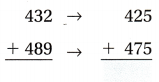So, about ________ dogs went to Pine Lake Dog Park during June and August.
Explanation:
In the month of June 432 dogs went to park
In the month of August 489 dogs went to park
When they are estimated close to an exact number
425 + 475 = 900
So, about 900 dogs went to Pine Lake Dog Park during June and August.

Math Talk
Mathematical Processes

Will the sum of the compatible numbers 425 and 475 be greater than or less than the exact sum? Explain.
Less then the exact sum
Explanation
425 + 475 = 900
432 + 489 = 921
the sum of the compatible numbers are less than the exact sum
So, 900 < 921

Question 1.
What other compatible numbers could you have used?
400 and 500
425 is nearer to 400 rounding to hundred and 475 is rounded to 500
Explanation:
Rounding to nearest hundreds can be used, to compete the numbers.

Question 2.
About how many dogs went to the park during July and August? What compatible numbers could you use to estimate?
Explanation:
According to the given information,
July month 317 dogs went to park
August month 489 dogs went to the park
The sum of the dogs is 806,
when rounded to nearest hundreds, it is 800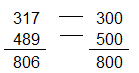Another Way Use place value to round.

432 + 489 = ☐
First, find the place to which you want to round. Round both numbers to the same place. The greatest place value of 432 and 489 is hundreds. Round each number to the nearest hundred.
400 + 500 = 900

Find the sum of the rounded numbers.
400 + 500 = ________
Explanation:
432 is rounded to the nearest hundred as 400
489 is rounded to the hundred as 500
So, 400 + 500 = 900

Math Talk
Mathematical Processes

How would you round 432 and 489 to the nearest ten? What would be the estimated Explain?
430 and 490
Explanation:
432 is rounded to nearest hundred is 430 and
489 is rounded to nearest hundred is 490

Share and Show

Estimate the sum.

Question 1.
Use compatible numbers.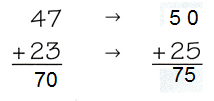Explanation:
The numbers which are close to the actual numbers, and can be easily added, subtracted or divided orally are known as compatible numbers.
47 is rounded to  nearest tens 50 and
23 is rounded to nearest tens 25

Question 2.
Use rounding.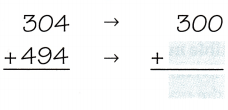Explanation:
The numbers which are close to the actual numbers, and can be easily added, subtracted or divided orally are known as compatible numbers.
304 is rounded to nearest hundred as 300
494 is rounded to nearest hundred as 500

Use rounding or compatible numbers to estimate the sum.

Question 3.Explanation:
The numbers which are close to the actual numbers, and can be easily added, subtracted or divided orally are known as compatible numbers.
Estimates means rounding to the nearest tens or hundreds.
421 can be estimated to a compatible number 420 and
218 can be written as 220 to nearest ten, then
the sum of the 420 + 220 = 640

Question 4.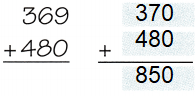Explanation:
The numbers which are close to the actual numbers, and can be easily added, subtracted or divided orally are known as compatible numbers.
Estimates means rounding to the nearest tens or hundreds.
369 can be estimated to a compatible number 370 and
480 is exactly rounded to its tens,
So, the sum of the 370 + 480 = 850

Question 5.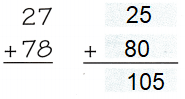Explanation:
The numbers which are close to the actual numbers, and can be easily added, subtracted or divided orally are known as compatible numbers.
Estimates means rounding to the nearest tens or hundreds.
27 can be estimated to a compatible number 25 and
78 can be written as 80 to nearest ten, then
the sum of the 25 + 80 = 105

Question 6.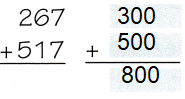Explanation:
The numbers which are close to the actual numbers, and can be easily added, subtracted or divided orally are known as compatible numbers.
Estimates means rounding to the nearest tens or hundreds.
267 can be estimated to a compatible number 300 and
517 can be written as 500 to nearest hundred, then
the sum of the 300+ 500 = 800

Question 7.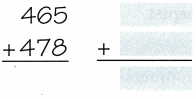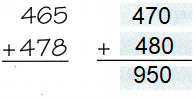Explanation:
The numbers which are close to the actual numbers, and can be easily added, subtracted or divided orally are known as compatible numbers.
Estimates means rounding to the nearest tens or hundreds.
465 can be estimated to a compatible number 470 and
478 can be written as 480 to nearest ten, then
the sum of the 470 + 480 = 950

Question 8.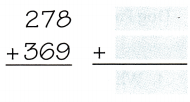Explanation:
The numbers which are close to the actual numbers, and can be easily added, subtracted or divided orally are known as compatible numbers.
Estimates means rounding to the nearest tens or hundreds.
278 can be estimated to a compatible number 280 and
369 can be written as 370 to nearest ten, then
the sum of the 280 + 370 = 650

Problem Solving

Question 9.
Multi-Step Green Lawn Service ordered 186 bags of top soil and 462 bags of fertilizer. Estimate to the nearest hundred how many bags Green Lawn Service ordered.Explanation:
Green Lawn Service ordered 186 bags of top soil and
462 bags of fertilizer.
estimating to the nearest hundred
186 is rounded to 200 and 462 to 500
So, 200 + 500 = 700

Problem Solving

Use the table for 10-13.Question 10.
About how many pet bowls were sold in June and July altogether?
148 pet bowls
Explanation:
pet bowls sold in the month of June = 91,
pet bowls sold in the month of July = 57
total pet bowls sold in both the months
91 + 57 = 148

Question 11.
How much bags of pet food sold in the month June and  July together? rounded nearest hundred?
Explanation:
Pet food sold in the month of June 419  and
Pet food sold in the month of July 370
Total food sold in both the months
419 + 370 = 789
when rounded to nearest hundreds
400 + 400 = 800

Question 12.
H.O.T. Multi-Step Dan estimated the lowest monthly sales of both pet bowls and bags of pet food to be about 300. What month had the lowest sales? Explain.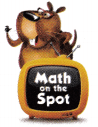Explanation:
From the above table the least sales month is August
The sales of pet bowls is 76
The sales of pet food bag is 228
the sum of pet bowl and bag of pet food
76 + 228 = 304
304 is rounded to 300

Question 13.
H.O.T. Multi-Step Dan said the total number of bags of pet food sold in June, July, and August was about 1,000. How did Dan estimate? Explain.
Explanation:
bags of pet food sold in June, July, and August
419 + 370 + 228 = 1017
1017 rounding to 1000, as 1017 is nearer to 1000

Fill in the bubble for the correct answer choice.

Question 14.
Use Tools The table shows the number of steps from bottom to top for each pyramid. Which of these statements shows the best estimate of how many steps there are in both pyramids?(A) 5 + 100 = 105
(B) 0 + 75 = 75
(C) 10 + 90 = 100
(D) 6 + 95 = 101
Option (C)
Explanation:
Number of steps in Djoser is 6
Number of steps in Chichen Itza is 91
The sum of both pyramids
6 + 91 = 97
write the estimates of both the sums
10 + 90 = 100
as 97 can be rounded to 100

Question 15.
Lea and Josh used sugar cubes to build a model of a pyramid. First, they glued 196 cubes to make a square base. Next, they used 144 cubes to make the first step. Which is the best estimate of the number of sugar cubes Lea and Josh used to build the first two steps of their pyramid?
(A) 440
(B) 300
(C) 220
(D) 400
Option (B)
Explanation:
First, they glued 196 cubes to make a square base.
Next, they used 144 cubes to make the first step.
The best estimate of the number of sugar cubes Lea and Josh used to build the first two steps of their pyramid
196 + 144 = 340
Estimating to compatible number 300

Question 16.
Multi-Step The height of the Great Pyramid of Giza is about 481 feet. The length of one side of its base is 755 feet. What is the best estimate of the sum of the height and length?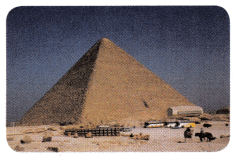(A) 1,000 feet
(B) 1,100 feet
(C) 1,300 feet
(D) 1,500 feet
Option (B)
Explanation:
The height of the Great Pyramid of Giza is about 481 feet.
The length of one side of its base is 755 feet.
The sum of both pyramids
481 + 755 = 1,236
The best estimate of the sum of the height and length 1,100

Texas Test Prep

Question 17.
Tracy ordered 325 toys and 165 bags of food for her new pet store. Which is the best estimate of the total number of items Tracy ordered?
(A) 700
(B) 500
(C) 400
(D) 600
Option (B)
Explanation:
Tracy ordered 325 toys and 165 bags of food for her new pet store.
The best estimate of the total number of items Tracy ordered
325 + 165 = 300 + 200 = 500

### Texas Go Math Grade 3 Lesson 4.2 Homework and Practice Answer Key

Use rounding or compatible numbers to estimate the sum.

Question 1.
18 + 53
20 + 50 = 70
Explanation:
The numbers which are close to the actual numbers, and can be easily added, subtracted or divided orally are known as compatible numbers.
18 is rounded to nearest ten as 20
53 is rounded to nearest ten as 50

Question 2.
37 + 52
40 + 50 = 90
Explanation:
The numbers which are close to the actual numbers, and can be easily added, subtracted or divided orally are known as compatible numbers.
37 is rounded to nearest ten as 40
52 is rounded to nearest ten as 50

Question 3.
345 + 213
350 + 200 = 550
Explanation:
The numbers which are close to the actual numbers, and can be easily added, subtracted or divided orally are known as compatible numbers.
345 is rounded to nearest ten as 350
213 is rounded to nearest ten as 200

Question 4.
509 + 478
500 + 480 = 980
Explanation:
The numbers which are close to the actual numbers, and can be easily added, subtracted or divided orally are known as compatible numbers.
509 is rounded to nearest ten as 500
478 is rounded to nearest ten as 480

Question 5.
542 + 212
540 +210 = 750
Explanation:
The numbers which are close to the actual numbers, and can be easily added, subtracted or divided orally are known as compatible numbers.
542 is rounded to nearest ten as 540
212 is rounded to nearest ten as 210

Question 6.
109 + 688
100 + 700 = 800
Explanation:
The numbers which are close to the actual numbers, and can be easily added, subtracted or divided orally are known as compatible numbers.
109 is rounded to nearest hundred as 100
688 is rounded to nearest hundred as 700

Problem Solving

Question 7.
Seth bought a pair of sneakers for $48 and a jacket for$64. Explain how you can estimate to find the total amount that he spent for the sneakers and jacket.
Answer: $112 Explanation: Seth bought a pair of sneakers for$48 and a jacket for $64. the total amount that he spent for the sneakers and jacket.$48 + $64 =$112
$112 can be rounded to$110 as compatible number to estimate.

Question 8.
Elena drove 245 miles last week and 222 miles this week. She estimated that she drove about 475 miles in both weeks. How did Elena estimate?
467 can be rounded to 475 as compatible number to estimate
Explanation:
Elena drove 245 miles last week and 222 miles this week.
She estimated that she drove about 475 miles in both weeks.
245 + 222 = 467
467 can be rounded to 475 as compatible number to estimate.

Lesson Check

Question 9.
Cal has $42. He gets$25 from his grandparents for his birthday. Which of these statements shows the best estimate of the amount of money Cal has now?
(A) $50 +$30 = $80 (B)$50 + $20 =$70
(C) $40 +$40 = $80 (D)$40 + $25 =$65
Option (B)
Explanation:
Cal has $42. He gets$25 from his grandparents for his birthday.
Total amount Cal has now
42 + 25 = 67
the best estimate of the amount of money Cal has now
67 can be rounded to 70 as compatible number to estimate.

Question 10.
Mr. Avery’s class made $118 for the fundraiser. Mrs. Bolero’s class made$189 for the fundraiser. Which of these statements shows the best estimate of the total amount both classes raised?
(A) $100 +$200 = $300 (B)$125 + $150 =$275
(C) $100 +$100 = $200 (D)$200 + $200 =$400
Option (A)
Explanation:
Mr. Avery’s class made $118 for the fundraiser. Mrs. Bolero’s class made$189 for the fundraiser.
The total amount of both classes raised
118 + 189 = 307
the best estimate of the total amount both classes raised
307 can be rounded to 300 as compatible number to estimate

Question 11.
Which is the best estimate of the total number of students in kindergarten and first grade at Wayside Elementary School?(A) 350
(B) 300
(C) 400
(D) 500
Option (C)
Explanation:
Number of students in Kindergarten = 187
Number of students in First grade = 203
The sum of the two classes
187 + 203 = 390
390 can be rounded to 400 as compatible number to estimate

Question 12.
Multi-Step Which is the best estimate of the total number of students in second, third, and fourth grade at Wayside Elementary School?
(A) 500
(B) 400
(C) 700
(D) 800
Option (D)
Explanation:
Students in Second class= 382
Students in Third class = 238
Students in Forth class = 178
382 + 238 + 178 = 798
798 can be rounded to 800 as compatible number to estimate

Question 13.
Multi-Step Which is the best estimate of the total number of students in all grades at Wayside Elementary School?
(A) 900
(B) 1,200
(C) 1,100
(D) 1,000